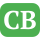CppBuzz.com

# Type Casting in C Programming

Type Casting is a way to convert one type of variable to another type. By type we mean Data Type (int, char, float etc..)

• Implicit
• Explicit

## Implicit type casting

Implicit type casting take place automatically. We don't need any operator for this type casting, however there are chances to loose some data type.

```#include <stdio.h>

void main () {
int a;
float b= 5.30;
a = b;
printf("%d", a);
}
```

If you look at the above example then we are converting float type to int type ie a = b; here a will store only 5 instead of storing 5.30; it becuase the .30 value is discarded becuase int data type can't store it. Since it happens automatically so it is known as implicit conversion.

Thumb Rule - Implicit casting can take place when converting from bigger data type to smaller data type and vice versa. We just need to keep in mind that if bigger data types implicitly converted to smaller data type (like in the above example ) then there may be loss of data. Here the converion is taking place from float to int (int is smaller data type than float; .30 is getting lost)

## Explicit type casting

Explicit type casting is achieved by forcing conversion of one type to another type. This type casting is achieved by "()" operator. Look at the below code, its output is 1.0. It is 1.0 because the int int division is int only. (float float division is float only)

```#include <stdio.h>

void main () {
int a,b;
float c;
a = 5; b = 3;

c = a/b;
printf("%f", c);
}
```

If we want the correct output then we have to explicitly cast it -

```
#include <stdio.h>

void main () {
int a,b;
float c;
a = 5; b = 3;

c = (float)a/b;
printf("%f", c);
}
```

(float) is used here with a; actually it can be used with with a or b, anyway would give the correct result.

Thumb Rule:- The result of division is biggest data types. In our example above int int division is int only so when we need the exact output which is as double then we have to make any one of operand as double

So, this was all about type casting in C programming. If you want to practice then you can play around with other data types.